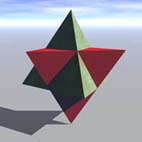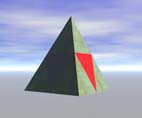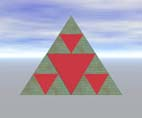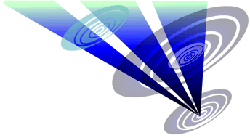Crop Circle Geometry
3 D - Fractals

 In the mid 90s some of the found crop circles were based on fractals. For instance the so-called 'Julia set' at Stonehenge in 1996 and the 'Triple Julia set' at Windmill Hill the same year. Or the Koch-fractals in 1997. In later years, this resembling disappeared and was replaced by other features. In 1999 many of the crop circles looked three-dimensional. But did the fractals really disappear?If you make a drawing of the 1999 West Overton  formation, cut it out and fold it together, you get  a three-dimensional shape: an octahedron. See image. iThis octahedron forms the core of a three-dimensional  fractal. To understand this we shall first have a look  at an other Platonic solid; the tetrahedron. See image.By taking a second tetrahedron and putting it upside  down 'in' the first tetrahedron, we get a so-called  star-tetrahedron. See image.When we take this star-tetrahedron and 'pull out'  the red tetrahedron, we are left with a tetrahedron  with its 'heart' taken out. The empty space in the  tetrahedron is where the two tetrahedrons were  overlapping. See image.This empty space we can fill up again.  See image.If we just look at the shape of the filling-up material,  we see an octahedron! With other words: the heart  of a star-tetrahedron is an octahedron. See images.The next image shows the tetrahedron with the  filling. You are looking at one side of the tetrahedron.  All the other sides look like this. What we see is an  upside down red equilateral triangle (=octahedron)  in a bigger equilateral triangle (=tetrahedron).We can repeat the same procedure with the three  green equilateral triangles, which are in fact three  tetrahedrons. The next image shows the result.  We are now looking at the second step of the  iteration that forms a Sierpinsky Gasket, which  is a well-known fractal!The procedure described above can be repeated  again and again forming a three-dimensional  Sierpinsky Gasket. A true three-dimensional fractal!  Remember: the red fillings are in fact octahedrons,  the shape formed by folding together the West  Overton 1999 formation.So the fractals did not disappear, at least not up  until 1999. They were just hidden. And more:  they were hidden in a three-dimensional form! (c) text and diagrams: Bert Janssen.##Bert Janssen & Janet Ossebaard